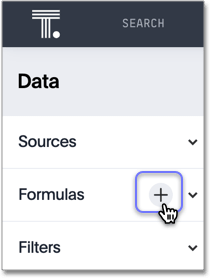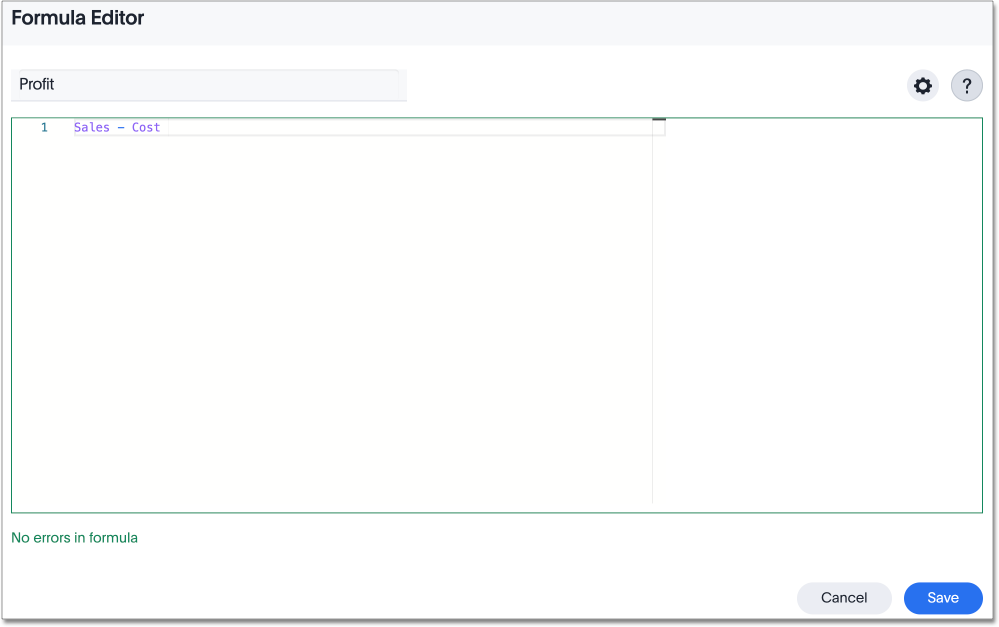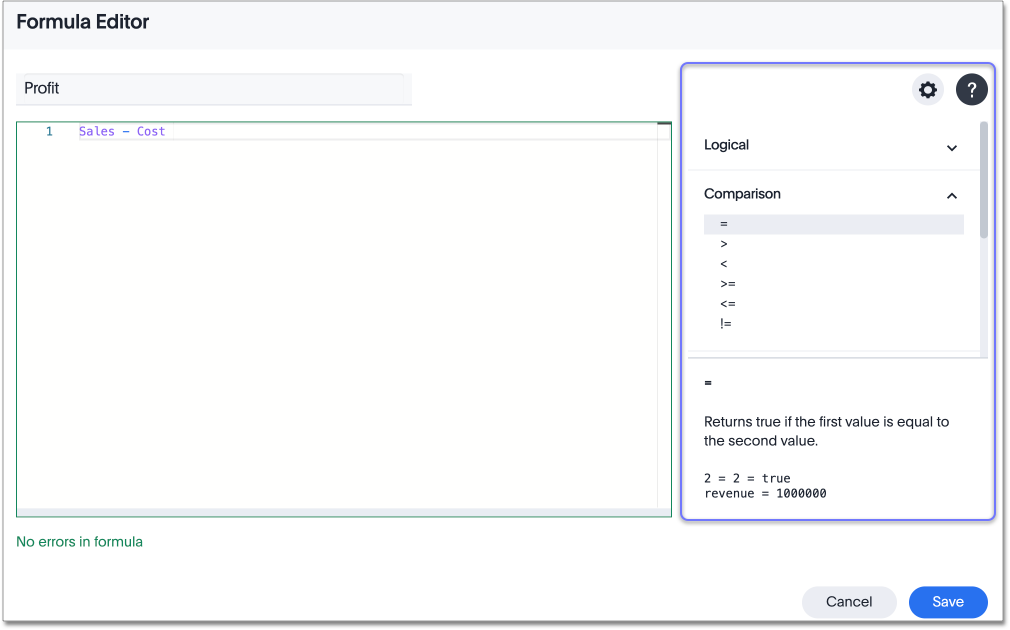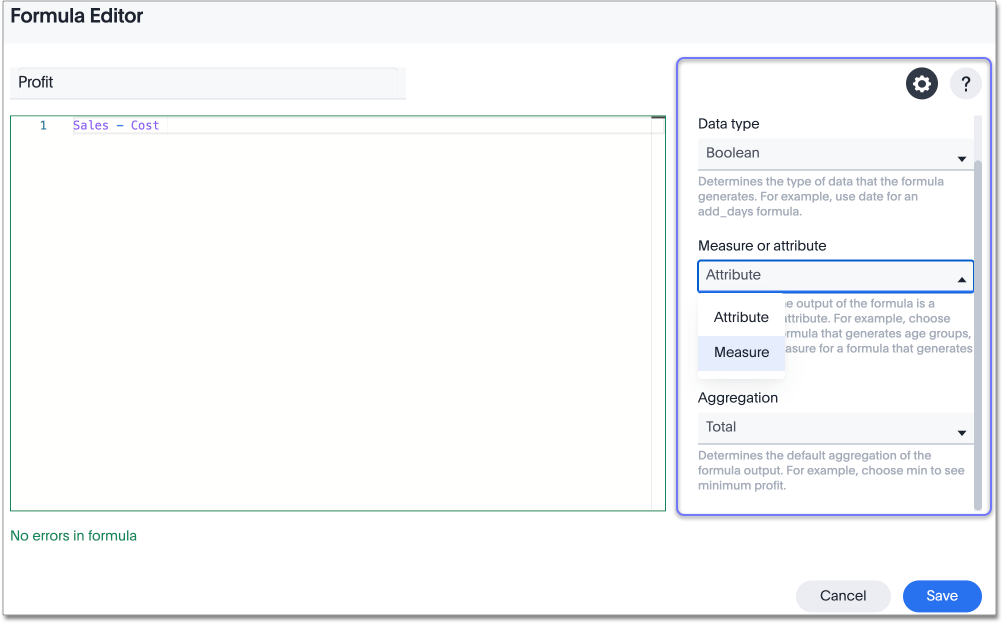Create a formula in a worksheet

You can define formulas and use them to create derived columns in worksheets.

To create formulas, combine standard functions and operators, column names, and constant values.

Anyone who can create a worksheet can add a formula to it. Formulas are not reusable; the formula you create is associated only with the worksheet it belongs to. A complete list of available formulas and examples of each is available in the Formula function reference.

You can create a formula in a worksheet by using the Formula Builder. When you do this, the result of the formula gets added to the worksheet as a column. Use these steps to create a formula:

1. Click the + button next to Formulas.2. Type your formula in the Formula Builder.Formula elements are color coded by type and can include the formula operators and functions (blue), the names of columns (purple), and/or constants (black).
3. You can see a list of formula operators with examples by clicking on Formula Assistant.4. If you want to change what your formula returns, use the Advanced settings.

Depending on your formula, you may be able to change:

• Data type

• ATTRIBUTE or MEASURE

• Aggregation type5. Name the formula by clicking on its title and typing the new name. Click Save.

Use aggregate formulas as Worksheet filters

Starting in ThoughtSpot release 6.2, you can use an aggregate formula as a Worksheet filter. This is useful when, for example, you only want your results to show a measure when the related attribute is greater than some number, or vice versa. You may only want to see sales when the unique customer count is greater than 1, or you may only want to see a customer if the associated sales is greater than 0. Rather than add that formula to every search, you can create a filter at the Worksheet level.